Here we are providing Class 12 Physics Important Extra Questions and Answers Chapter 8 Electromagnetic Waves. Important Questions for Class 12 Physics with Answers are the best resource for students which helps in Class 12 board exams.

## Class 12 Physics Chapter 8 Important Extra Questions Electromagnetic Waves

### Electromagnetic Waves Important Extra Questions Very Short Answer Type

Question 1.
Name the part of the electromagnetic spectrum which has the longest wavelength and write its one use. (CBSE 2019C)

• In the electromagnetic spectrum, long radio waves have the longest wavelength.
• Radio waves are used in communication systems.

Question 2.
The small ozone layer on the top of the stratosphere is crucial for human survival. Why?
The ozone layer absorbs the ultraviolet rays, emitted by the sun, which are harmful to the living tissues of human beings.

Question 3.
Name the part of the electromagnetic spectrum which is used in the “greenhouse” to keep plants warm.
Infrared rays.

Question 4.
How are radio waves produced? (CBSE AI 2011)
They are produced by rapid acceleration and decelerations of electrons in aerials.

Question 5.
How are X-rays produced? (CBSE Al 2011)
By the transition of inner-shell electrons.

Question 6.
How are microwaves produced? (CBSE AI 2011)
By using a magnetron.

Question 7.
A plane electromagnetic wave travels in a vacuum along the z-direction. What can you say about the direction of electric and magnetic field vectors? (CBSE Delhi 2011)
The electric and magnetic field vectors will be along the x and y directions.

Question 8.
What is the frequency of electromagnetic waves produced by the oscillating charge of frequency v? (CBSE Delhi 2011C)
The frequency of electromagnetic waves produced by the oscillating charge of frequency v is also v.

Question 9.
What are the directions of electric and magnetic field vectors relative to each other and relative to the direction of propagation of electromagnetic waves? (CBSE AI 2012)
The three are mutually perpendicular to one other.

Question 10.
Welders wear special goggles or face masks with glass windows to protect their eyes from electromagnetic radiation. Name the radiations and write the range of their frequency. (CBSE Al 2013)
UV radiations, 1015 to 1017 Hz

Question 11.
To which part of the electromagnetic spectrum does a wave of frequency 5 × 1019 Hz belong? (CBSEAI 2014)
X – rays

Question 12.
The available frequency AC source is connected to a capacitor. Will the displacement current change if the frequency of the AC source is decreased? (CBSE Al 2015C)
No

Question 13.
Why microwaves are considered suitable for radar systems used in aircraft navigation? (CBSE Delhi 2016)
They have a small wavelength and travel along a straight line with deflecting.

Question 14.
Do electromagnetic waves carry energy and momentum? (CBSE AI 2017)
Yes.

Question 15.
How is the speed of em-waves in vacuum – determined by the electric and magnetic field? (CBSE Delhi 2017)
c = $$\frac{E_{0}}{B_{0}}$$

Question 16.
Why is skywave propagation of signals restricted to a frequency of 30 MHz? (CBSE Al 2017 C)
The atmosphere is transparent to frequencies higher than 30 MHz.

Question 17.
Name the electromagnetic radiations used for
(a) water purification

(b) eye surgery. (CBSEAI 2018, Delhi 2018)
Visible light

Question 18.
Write the range of frequencies of electromagnetic waves which propagate through sky wave mode. (CBSE Al 2018 C)
A few MHz up to 30 to 40 MHz.

Question 19.
Optical and radio telescopes are built on the ground but X-ray astronomy is possible only from satellites orbiting the earth. Why?
This is because of the fact that X-rays are absorbed by the atmosphere, whereas light and radio waves penetrate through it.

Question 20.
A charged particle oscillates about its mean equilibrium position with a frequency of 109 Hz. What is the frequency of the electromagnetic wave produced by the oscillator?
Same as that of the oscillating charged particle, i. e. 109 Hz.

Question 21.
Why are infrared radiations referred to as heat waves also? Name the radiations which are next to these radiations in the electromagnetic spectrum having
(a) Shorter wavelength and
(b) Longer wavelength.
This is because they produce a heating effect.
(a) Visible light and
(b) Microwaves.

Question 22.
What physical quantity are the same for X-rays of wavelength 10-10 m, the red light of wavelength 680 nm, and radio waves of wavelength 500 m?
Since all of them are electromagnetic waves, their speed in a vacuum will be the same, i.e. 3 × 108 m s-1.

Question 23.
A charged particle oscillates about its mean equilibrium position with a frequency of 109 Hz. What is the frequency of the electromagnetic waves produced by the oscillator?
The frequency of the electromagnetic waves produced by the oscillator is the same as its frequency of vibration, i.e. 109 Hz.

Question 24.
The amplitude of the magnetic field part of a harmonic electromagnetic wave in a vacuum is B0 = 510 nT. What is the amplitude of the electric field part of the wave?
Given B0 = 510 nT = 510 × 10-9 T, E0 = ?

Using the relation E0 = c × B0, we have
E0 = 3 × 108 × 510 × 10-9 = 153 N C-1

Question 25.
Why does a microwave oven heat up a food item containing water molecules most efficiently?
It is because the frequency of the microwave matches the resonant frequency of water molecules. This makes the molecules vibrate with maximum amplitude thereby producing heat.

Question 26.
Name the most energetic electromagnetic radiation and write its frequency range. (CBSE AI 2019 C)
The most energetic radiations are Gamma Rays Frequency range of gamma rays is: 1018 Hz to 1023 Hz
Or
Name the electromagnetic radiations used in eye surgery or to kill germs in water purifiers. Write its frequency, range.

1. Radiations used for eye surgery or to kill germs are Ultraviolet Rays.
2. The frequency range of ultraviolet rays: 105 Hz to 1017 Hz.

### Electromagnetic Waves Important Extra Questions Short Answer Type

Question 1.
Radio waves and gamma rays both are transverse in nature and electromagnetic in character and have the same speed in a vacuum. In what respect are they different?
The radio waves have an atomic origin, while gamma rays have a nuclear origin. Further owing to their very small wavelength, gamma rays are highly penetrating in comparison to radio waves.

Question 2.
Show that the average energy density of the electric field equals the average density of the magnetic field.
The average density of the electric field is given by
Ue = $$\frac{1}{2}$$ε0E2 and the average energy density of the magnetic field is given by UB = $$\frac{B^{2}}{2 \mu_{0}}$$.

But B = $$\frac{E}{c}$$ and c = $$\frac{1}{\sqrt{\mu_{0} \varepsilon_{0}}}$$ , hence the above equation becomes UB = $$\frac{B^{2}}{2 \mu_{0}}=\frac{E^{2}}{2 \mu_{0} c^{2}}$$

UB = $$\frac{E^{2}}{2 \mu_{0} \times \frac{1}{\mu_{0} \varepsilon_{0}}}=\frac{1}{2} \varepsilon_{0} E^{2}$$. Hence the result.

Question 3.
State four properties of electromagnetic waves.
(a) They do not require any material medium to travel.
(b) They are transverse in nature, i.e. electric and magnetic fields are perpendicular to each other and also to the direction of the propagation of the wave.
(c) The energy of the wave is divided equally amongst the electric and the magnetic field.
(d) They travel, in free space, with a velocity of 3 × 108 m s-1.

Question 4.
(a) λ1 are used to kill germs in water purifiers.
(b) λ2 are used in TV communication systems.
(c) λ3 plays an important role in maintaining the earth’s warmth.
Name the part of the electromagnetic spectrum to which these radiations belong. Arrange these wavelengths in decreasing order of their magnitude.
(b) λ2 – Microwaves
(c) λ3 – Infrared rays
Their order is λ1 < λ3 < λ2.

Question 5.
Name the constituent radiation of the electromagnetic spectrum which
(a) is used in satellite communication.
Microwaves.

(b) is used for studying crystal structure.
X-rays

(c) is similar to the radiations emitted during the decay of a radioactive nucleus.
Gamma rays

(d) is absorbed from sunlight by the ozone layer.
UV rays

(e) produces an intense heating effect.
Infrared rays

(f) has its wavelength range between 390 nm and 770 nm.
Visible light.

Question 6.
Name the radiations of the electromagnetic spectrum which are used in
(a) warfare to look through the haze.
Infrared rays

Microwaves.

(c) studying the structure and properties of atoms and molecules.
Gamma rays.

Question 7.
Why are microwaves used in RADAR?
Microwaves are electromagnetic waves of very short wavelength. Such waves are used in RADAR due to the reason that they can travel in a particular direction in the form of a beam without being deflected.

Question 8.
Electromagnetic waves with wavelength
(a) λ1 are used to treat muscular strain.
(c) λ3 are used to detect fractures in bones.
(d) λ4 are absorbed by the ozone layer of the atmosphere.
Identify and name the part of the electromagnetic spectrum to which these radiations belong. Arrange
these wavelengths in decreasing order of magnitude.
(a) Infrared radiations are used to treat muscular strain.
(c) X-rays are used to detect fractures in bones.
(d) Ultraviolet radiation is absorbed by the ozone layer of the atmosphere.
The decreasing order of their wavelength is
λ2 > λ1 > λ4 > λ3.

Question 9.
(a) Draw a graph of a linearly polarised em wave propagating in the Z-direction showing the directions of the oscillating electric and magnetic fields.
Graph of linearly polarised em wave propagating in the Z-axis.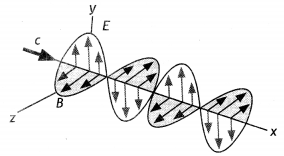(b) Write the relations (i) between the speed of light and the amplitudes of electric and magnetic fields, (ii) for the speed of em wave in terms of a permittivity e0, and magnetic permeability p0, of the medium. (CBSE 2019C)
(i) Relation between speed of light and amplitudes of electric and magnetic field c = $$\frac{E_{0}}{B_{0}}$$

(ii) Speed of light in terms of ε<sub>0</sub> and μ0,
c = $$\frac{1}{\sqrt{\mu_{0} \varepsilon_{0}}}$$

Question 10.
Arrange the following electromagnetic waves in the order of their increasing wavelength:
(a) Gamma rays
(b) Microwaves
(c) X-rays
How are infrared waves produced? What role does infrared radiation play in
(a) maintaining the Earth’s warmth and
(b) physical therapy? (CBSE Al 2015)
Gamma(γ) rays, X-rays, Microwaves, Radio waves:
Infrared rays are produced by hot bodies/ vibration of atoms and molecules Infrared rays: (a) Maintain the earth’s warmth through the greenhouse effect, (b) produce heat

Question 11.
Name the parts of the electromagnetic spectrum which is
Microwave: They are produced by oscillating circuits.

(b) used to treat muscular strain.
Infrared rays: They are produced by the vibration of atoms and molecules.

(c) used as a diagnostic tool in medicine. Write in brief, how these waves can be produced. (CBSE Delhi 2015)
X-rays: They are produced by bombarding high atomic number targets with electrons.

Question 12.
An e.m wave Y1 has a wavelength of 1 cm while another e.m wave, Y2 has a frequency of 1015 Hz. Name these two types of waves and write one useful application for each. (CBSE AI 2016 C)
Y1 – Microwaves and
Y2 – Ultraviolet waves.

• Microwaves: used for communication.
• Ultraviolet waves: used for sterilization.

Question 13.
Identify the electromagnetic waves whose wavelengths vary as
(a) 10-12 m < λ < 10-8 m
X – rays: a study of crystal structure

(b) 10-3 m < λ < 10-1 m. Write one of their uses. (CBSE Al 2017)

Question 14.
Name the type of e.m waves having a wavelength range of 0.1 m to 1 mm. How are these waves generated? Write their two uses. (CBSE Al 2017 C)

• Microwaves: These are generated with the help of special vacuum tubes (called klystrons, magnetrons, and Gunn diodes).
• Uses: Cooking, radar, and communication

Question 15.
(a) Give one use of electromagnetic radiations obtained in nuclear disintegrations.
Treating cancer.

(b) Give one example each to illustrate the situation where there is
(i) displacement current but no conduction current and
Between the plates of a capacitor

(ii) only conduction current but no displacement current.(CBSE Al 2018 C)
Outside the plates of a capacitor

Question 16.
Scientists predict that a global nuclear war on the earth will be followed by a severe nuclear winter, with devastating effects on the earth. What is the basis of this prediction?
The explosions will produce so much dust, which will cover the whole atmosphere, thereby blocking the sun’s rays from reaching the earth. This will cause the setting in of a long winter, which is called nuclear winter.

Question 17.
If the earth did not have an atmosphere, would its average surface temperature be higher or lower than what it is now?
Due to the Greenhouse effect, the temperature of the earth’s surface is raised in the presence of the atmosphere. In the absence of the atmosphere, the heat received by the earth during the day is completely lost during the night. Hence the average surface temperature will be lower than the preset temperature.

Question 18.
A capacitor of capacitance ‘C is being charged by connecting it across a dc source along with an ammeter. Will the ammeter show a momentary deflection during the process of charging? If so, how would you explain this momentary deflection and the resulting continuity of current in the circuit? Write the expression for the current inside the capacitor. (CBSE AI 2012)
Yes, this is due to the rate of change of electric flux inside the capacitor due to the production of displacement current. The expression for the current inside the capacitor is lD = εo $$\frac{d \phi_{E}}{d t}$$

Question 19.
Define displacement current. What role does it play while charging a capacitor by dc source? Is the value of displacement current the same as that of the conduction current? Explain. (CBSE AI 2019)

• Displacement current is the current due to the change of electric flux.
• It provides continuity of current in circuits containing capacitors.
• Yes, the value of displacement current is equal to the conduction current.
• ld = εo $$\frac{d \phi_{e}}{d t}$$

Question 20.
Why is the orientation of the portable radio with respect to the broadcasting station important? (NCERT Exemplar)
This is because the electromagnetic waves are plane polarised; hence the receiving antenna should be parallel to the electric/magnetic part of the wave.

Question 21.
(a) Give one use of electromagnetic radiations obtained in nuclear disintegrations.
used to destroy cancer cells

(b) Give one example each to illustrate the situation where there is
(i) displacement current but no conduction current and
The region, between the plates of a capacitor, connected to a time-varying voltage source, has a displacement current but no conduction current.

(ii) only conduction current but no displacement current. (CBSE Delhi 2018C)
The wires, connected to the plates of a capacitor, joined to a time-varying or steady voltage source, carry a conduction current but no displacement current. (Alternatively, A circuit, having no capacitor in it, and carrying a current has conduction current but no displacement current.)

### Electromagnetic Waves Important Extra Questions Long Answer Type

Question 1.
(a) Name the em waves which are used for the treatment of certain forms of cancer. Write their frequency range.
Gamma rays.
Frequency range > 3 × 1020 Hz

(b) Thin ozone layer on top of the stratosphere is crucial for human survival. Why?
The thin ozone layer on top of the stratosphere is crucial for human survival because it absorbs most of the ultraviolet rays coming from the sun. If the ozone layer had not been there, then ultraviolet rays would have entered the earth and caused danger to the survival of the human race.

(c) An em wave exerts pressure on the surface on which it is incident. Justify. (CBSE Delhi 2014)
An em wave carries a linear momentum with it. The linear momentum carried by a portion of a wave having energy U is given by p = U/c.

Thus, if the wave incident on a material surface is completely absorbed, it delivers energy U and momentum p = U/c to the surface. If the wave is totally reflected, the momentum delivered is p = 2U/c because the momentum of the wave changes from p to – p. Therefore, it follows that an em wave incident on a surface exerts a force and hence a pressure on the surface.

Question 2.
(a) Why is the thin ozone layer at the top of the stratosphere crucial for human survival? Identify to which part of the electromagnetic spectrum does this radiation belongs and write one important application of the radiation.
The thin ozone layer on top of the stratosphere is crucial for human survival because it absorbs most of the ultraviolet rays coming from the sun. If the ozone layer had not been there, then ultraviolet rays would have entered the earth and caused danger to the survival of the human race. This radiation is UV radiation. It is used in sterilization.

(b) Why are infrared waves referred to as heat rays? How are they produced? What role do they play in maintaining the earth’s warmth through the greenhouse effect? (CBSE Delhi 2015C)
Infrared radiations heat up the material on which they fall, hence they are also called heat rays. They are produced by the vibration of atoms and molecules. After falling on the earth, they are reflected back into the earth’s atmosphere. The earth’s atmosphere does not allow these radiations to pass through as such they heat up the earth’s atmosphere.

Question 3.
How are electromagnetic waves produced? What is the source of energy of these waves? Write mathematical expressions for electric and magnetic fields of an electromagnetic wave propagating along the z-axis. Write any two important properties of electromagnetic waves. (CBSE AI 2016)
Electromagnetic waves are produced by accelerated charges which produce an oscillating electric field and magnetic field (which regenerate each other).

• Source of the Energy: Energy of the accelerated charge or the source that accelerates the charges.
• Expression: Ex = Eo sin (kz – ωt) and By = Bo sin (kz – ωt)
(a) They are transverse in nature.
(b) They don’t require a medium to propagate.

Question 4.
How are em waves produced by oscillating charges?
Draw a sketch of linearly polarised em waves propagating in the Z-direction. Indicate the directions of the oscillating electric and magnetic fields. (CBSE Delhi 2016)
(a) An oscillating charge produces an oscillating electric field in space, which produces an oscillating magnetic field. The oscillating electric and magnetic fields regenerate each other, and this results in the production of em waves in space.
(b) See Figure.Question 5.
Write Maxwell’s generalization of Ampere’s Circuital Law. Show that in the process of charging a capacitor, the current produced within the plates of the capacitor is i = εo$$\frac{d \phi_{E}}{d t}$$ where ΦE is the electric flux produced during charging of the capacitor plates. (CBSE Delhi 2016)
The generalized form of Maxwell ampere law is
$$\oint \vec{B} \cdot \overrightarrow{d l}$$= μo(l + lD) where lD = εo$$\frac{d \phi_{E}}{d t}=\frac{d q}{d t}$$

The electric flux Φ between the plates of the parallel plate capacitor through which a time-dependent current flow is given by:
ΦE = E A, but E = σ/εo
Therefore we haveQuestion 6.
(a) Why are Infrared waves often called heatwaves? Explain.
Infrared waves have frequencies lower than those of visibLe Light; they have the ability to vibrate not only the electrons but the entire atoms or molecules of a body. This vibration increases the internal energy and temperature of the body. That is why infrared waves are often called heat waves.

(b) What do you understand by the statement, “Electromagnetic waves transport momentum”? (CBSE AI, Delhi 2018)
If we consider a plane perpendicular to the direction of propagation of the electromagnetic wave, then electric charges present on the plane will be set and sustained in motion by the electric and magnetic fields of the electromagnetic wave. The charges present on the surface thus acquire energy and momentum from the waves. This just illustrates the fact that an electromagnetic wave (like other waves) transfers energy and momentum.

Question 7.
(a) When the oscillating electric and magnetic fields are along the x- and y-direction respectively
(i) point out the direction of propagation of the electromagnetic wave,
Z-axis

(ii) express the velocity of propagation in terms of the amplitudes of the oscillating electric and magnetic fields.
c = Eo / Bo

(b) How do you show that the em wave carries energy and momentum? (CBSE A! 2013C)
Consider a plane perpendicular to the direction of propagation of the electromagnetic wave. If there are, on this plane, electric charges, they will be set and sustained in motion by the electric and magnetic fields of the electromagnetic wave. The charges thus acquire energy and momentum from the waves. This illustrates the fact that an electromagnetic wave carries energy and momentum.

Question 8.
(a) Show, by giving a simple example, how em waves carry energy and momentum.
Consider a plane perpendicular to the direction of propagation of the electromagnetic wave. If there are, on this plane, electric charges, they will be set and sustained in motion by the electric and magnetic fields of the electromagnetic wave. The charges thus acquire energy and momentum from the waves. This just illustrates the fact that an electromagnetic wave (like other waves) carries energy and momentum.

(b) How are microwaves produced? Why is it necessary in microwave ovens to select the frequency of microwaves to match the resonance frequency of water molecules?
Microwaves (short-wavelength radio waves), with frequencies in the gigahertz (GHz) range, are produced by special vacuum tubes (called klystrons, magnetrons, and Gunn diodes). In such ovens, the frequency of the microwaves is selected to match the resonant frequency of water molecules so that energy from the waves is transferred efficiently to the kinetic energy of the molecules. This raises the temperature of any food containing water.

(c) Write two important uses of infrared waves. (CBSE Delhi 2014C)
Remote control of electronic devices, heating

Question 9.
A parallel plate capacitor is being charged by a time-varying current. Explain briefly how Ampere’s circuital law is generalized to incorporate the effect due to the displacement current. (CBSE AI 2011)
Consider the curve C bounding two surfaces S1 and S2. For the bound surface S1; the current passes through it, as such, we can write Ampere’s circuital law as
$$\oint_{s_{1}} \vec{\phi} \cdot \vec{d}$$ = μol …(1)

If however, we choose the bound surface S2 that passes through the plates of the capacitor and is not pierced by a current-carrying conductor, then Ampere’s circuital law is written as
$$\oint_{s_{2}} \vec{\phi} \cdot \vec{d}$$ = 0 ….(2)The above two equations contradict each other. To resolve this contradiction, Maxwell showed that this inconsistency is due to the assumed discontinuity of the current. According to Maxwell a current called displacement current “flows” between the plates of the capacitor, where there is no conduction current.

Therefore Ampere’s circuital law takes the generalised expression $$\oint \vec{B} \cdot d \vec{l}$$ = μo (l + lD),

here l is the conduction current and lD is the displacement current given by
lD = εo $$\frac{d \phi_{E}}{d t}=\frac{d q}{d t}$$.

Outside the plates of the capacitor, conduction current flows and displacement current is zero, whereas inside the plates of the capacitor, displacement current exists and there is no conduction current.

Question 10.
(a) Identify the part of the electromagnetic spectrum used in (i) radar and (ii) eye surgery. Write their frequency range.
Microwaves:
Frequency range (1010 to 1012 Hz) Ultraviolet rays:
Frequency range (1015 to 1017 Hz)

(b) Prove that the average energy density of the oscillating electric field is equal to that of the oscillating magnetic field. (CBSE Delhi 2019)
The average density of the electric field is
uE = $$\frac{1}{2}$$εo E2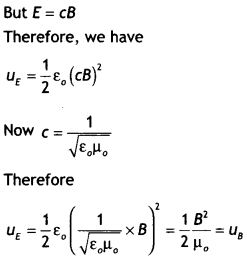Question 11.
The long-distance radio broadcast is not possible using long or medium wave bands because these waves, traveling as ground waves, can cover a maximum distance of 200 km. When used as sky waves, the short waves pass through the lower portion of the atmosphere but are reflected back from the ionosphere. In this way, short waves can travel very large distances and can even travel around the earth.

(b) It is necessary to use satellites for long-distance TV transmission. Why?
TV waves have a frequency range of 47 MHz to 940 MHz. These frequencies are not reflected by the ionosphere. As space waves, they can cover a distance of 50-60 km only. Therefore, for long-distance TV transmission, we make use of satellites that reflect the TV signal wave back towards the earth.

(c) Optical and radio telescopes are built on the ground but X-ray astronomy is possible only from satellites orbiting the earth. Why?
In optical and radio-telescopes we use visible light and radio waves, respectively, which can pass through the atmosphere. Hence, such telescopes are built on the ground. However, X-rays have extremely small wavelengths and are absorbed by the atmosphere. Hence, X-ray astronomy is not possible from ground stations. X-ray astronomy is possible only from satellites orbiting the earth at a height of 500 km or more.

(d) The small ozone layer on top of the stratosphere is crucial for human survival. Why?
The thin ozone layer on top of the stratosphere is crucial for human survival because it absorbs most of the ultraviolet rays coming from the sun. If the ozone layer had not been there, then ultraviolet rays would have entered the earth and caused danger to the survival of the human race.

(e) If the earth did not have an atmosphere, would its average surface temperature be higher or lower than what it is now?
If the earth did not have an atmosphere, then its average surface temperature would be lesser than what it is now because in that case greenhouse effect will be absent.

(f) Some scientists have predicted that a global nuclear war on the earth would be followed by a severe nuclear winter’ with a devastating effect on life on the earth. What might be the basis of this prediction?
The prediction is based on the assumption that the large dust clouds produced by global nuclear war would perhaps cover a substantial part of the sky and solar radiations will not be able to reach the earth. It may cause a severe winter on the earth with a devastating effect on life on the earth.

Numerical Problems

Question 1.
The frequency values v1 and v2, for two spectral lines of the e.m. spectrum are found to be 5 × 1020 Hz and 2.5 × 1011 Hz respectively. Find the ratio, λ12 of their wavelengths.Question 2.
In a plane electromagnetic wave, the electric field oscillates with a frequency of 2 × 1010 Hz and an amplitude of 40 VM.
(i) What is the wavelength of the wave and
(ii) what is the energy density due to the field?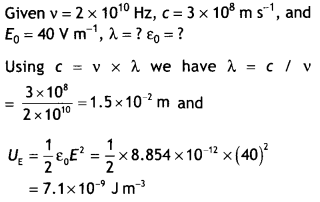Question 3.
A plane electromagnetic wave of frequency 25 MHz travels In free space along the x-direction. At a particular point In space and time, the electric vector is $$\vec{E}$$ = 6.3 Vm-1 ĵ. Calculate $$\vec{B}$$ at this point. (NCERT)
The magnitude of B and E are related as c = $$\frac{E}{B}$$, therefore B = $$\frac{E}{c}=\frac{6.3}{3 \times 10^{8}}$$ = 2.1 × 10-8. This field is along the z-axis, i.e. perpendicular to both the propagation of the wave and the electric field.

Question 4.
The magnetic field in a plane electromagnetic wave Is given by B = 2 × sin (0.5 × 10-3 x + 1.5 × 1011 t) T
(a) What are the wavelength and frequency of the wave?
(a) Comparing the given equation with the equation(b) Write an expression for the electric field. (NCERT)
Eo = cBo = 2 × 107 × 3 × 108 = 60 Vm-1

The electric field component is perpendicular to the direction of propagation and the direction of the magnetic field. Therefore, the electric field component aLong the z-axis is obtained as
Ez = 60 sin(0.5 × 103 × + 1.5 × 1011 t)Vm-1

Question 5.
A radio can tune In to any station in the 7.5 MHz to 12 MHz bands. What is the corresponding wavelength band? (NCERT)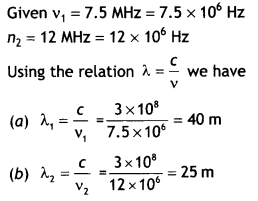Thus the wavelength band is 40 m to 25m.

Question 6.
Suppose that the electric field amplitude of an electromagnetic wave E0 = 120 N C-1 and that Its frequency is v = 50.0 MHz. (a) Determine, B0, ω k, and λ. (b) Find expressions for E and B. (NCERT)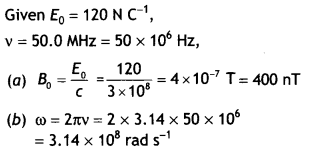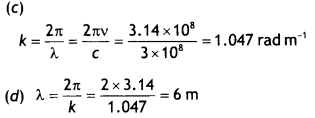Question 7.
In a plane electromagnetic wave, the electric field oscillates sinusoidally at a frequency of 2.0 × 1010 Hz and amplitude 48 V m-1.
(a) What is the wavelength of the wave?
(b) What is the amplitude of the oscillating magnetic field?
(c) Show that the average energy density of the E field equals the average energy density of the 8 fields. [c = 3 × 108ms-1.] (NCERT)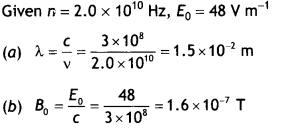(c) The average density of electric field is
given by Ue = $$\frac{1}{2}$$ε0E2 and the average energy density of the magnetic field is given by UB = $$\frac{B^{2}}{2 \mu_{0}}$$. But B = $$\frac{E}{c}$$ and C = $$\frac{1}{\sqrt{\mu_{0} \varepsilon_{0}}}$$ , hence the above equation becomes UB = $$\frac{B^{2}}{2 \mu_{0}}=\frac{E^{2}}{2 \mu_{0} c^{2}}$$,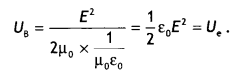Question 8.
Suppose that the electric field part of an electromagnetic wave in a vacuum is
E = {(3.1 NC-1>)cos(1.8 rad m-1)y + (5.4 × 106 rad s-1)t}î
(a) What is the direction of propagation?
(b) What is the wavelength?
(c) What is the frequency v?
(d) What is the amplitude of the magnetic field part of the wave?
(e) Write an expression for the magnetic field part of the wave. (NCERT)$$\vec{B}$$ = Bo cos (ky + ωt) k̂
$$\vec{E}$$ = {(10.3 n T)cos[(1.8 rad m-1)y + (5.4 × 106 rad s-1)t} î